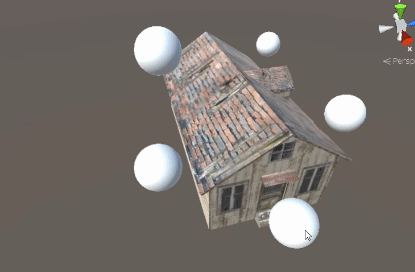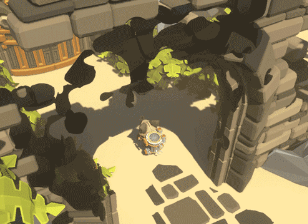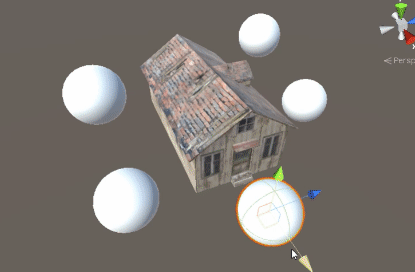# How can I dissolve using dynamic mask objects?

as you can see minions projected light on house to burning it out.

I like to know how to make a similar effect.• that picture is awesome! Sep 7, 2019 at 17:08

you can make this effect by dissolve in world space.

(I explained the world space in this post)

# Masking Objects by World Spaceyou can make array of positions and sizes in shader and use world space to fade them.

we also have to signal to the shader that these variables will be modified from outside, hence the uniform qualifier. As it happens in C, there is an extra variable which indicates how many points are actually used.

Shader "Smkgames/worldSpaceFade" {
Properties {
_Color ("Color", Color) = (1,1,1,1)
_MainTex ("Albedo (RGB)", 2D) = "white" {}
_NormalMap("Normal Map",2D) = "bump" {}
_Glossiness ("Smoothness", Range(0,1)) = 0.5
_Metallic ("Metallic", Range(0,1)) = 0.0
}
Tags {"Queue"="Transparent" "IgnoreProjector"="True" "RenderType"="Transparent"}
Pass {
ZWrite On
}
LOD 200

CGPROGRAM

#pragma target 3.0

sampler2D _MainTex;
sampler2D _NormalMap;

struct Input {
float2 uv_MainTex;
float3 worldPos: TEXCOORD2; // using world position
};

half _Glossiness;
half _Metallic;
fixed4 _Color;

void vert (inout appdata_full v, out Input o){
UNITY_INITIALIZE_OUTPUT(Input,o);
o.worldPos = mul(unity_ObjectToWorld, v.vertex); // calculating world position in vertex shader
}

uniform float4 _arrayPosition ; // declare array position
uniform float _arraySize ; // declare array size

UNITY_INSTANCING_BUFFER_START(Props)
UNITY_INSTANCING_BUFFER_END(Props)

void surf (Input IN, inout SurfaceOutputStandard o) {
fixed4 c = tex2D (_MainTex, IN.uv_MainTex) * _Color;

float4 color = float4(0,0,0,0); // temp color for using in alpha

for(int i = 0; i < 5;i++){
float dist = distance(IN.worldPos,
float4(_arrayPosition[i].xyz, 1.0)); // calculate distance in world position

if (dist < _arraySize[i]) // if distance is smaller than size of each object result is white in mask
{
color += float4(1.0, 1.0, 1.0, 1.0); // sum result of dynamic masks
}
}

o.Albedo = c.rgb;
o.Metallic = _Metallic;
o.Smoothness = _Glossiness;
o.Alpha =  saturate(1-color); // saturate to make sure is between 0 and 1 that is equivalent of clamp(value,0,1)
o.Normal = UnpackNormal(tex2D(_NormalMap,IN.uv_MainTex));
}
ENDCG
}
FallBack "Diffuse"
}


then you should initialize the positions and sizes of objects in shader:

using System;
using System.Collections;
using System.Collections.Generic;
using UnityEngine;

[ExecuteInEditMode]
{
public Transform[] arrayTransform;
public Vector4[] arrayPosition;
public float[] arraySize;
public Material material;

private void Awake()
{
arrayPosition = new Vector4[arrayTransform.Length];
arraySize = new float[arrayTransform.Length];
}

private void Update()
{
for (int i = 0; i < arrayTransform.Length; i++)
{
arrayPosition[i]= arrayTransform[i].position;
arraySize[i]= arrayTransform[i].localScale.x;

}

material.SetVectorArray("_arrayPosition",arrayPosition);
material.SetFloatArray("_arraySize",arraySize);
}
}


# Making World Position Dissolve Shader

I found a good tutorial that explained how to Dissolving Objects in the world:Dissolving the world Part 1

Dissolving the world Part 2Dissolving the world Chris Chen

Final Result:Shader "Smkgames/WorldDissolve" {
Properties {
_Color ("Color", Color) = (1,1,1,1)
_MainTex ("Albedo (RGB)", 2D) = "white" {}
_NormalMap("Normal Map",2D) = "bump" {}
_Glossiness ("Smoothness", Range(0,1)) = 0.5
_Metallic ("Metallic", Range(0,1)) = 0.0

_DissolvePoint("Dissolve point", Vector) = (0,0,0,0)
_DissolveAmount("Disolve amount", Range(0,1)) = 1
_MaxDistance("Max distance", float) = 1
_Intensity("Intensity", Range(1,5)) = 1
_NoiseFreq("Noise frequency", float) = 1
_Border("Border size", float) = 0.1
[HDR]_BorderColor("Border color", Color) = (1,0,0,1)
[HDR]_BorderEmission("Border emission", Color) = (1,0,0,1)
}
Tags {
"RenderType"="TransparentCutout"
"Queue"="AlphaTest"
}
Cull Off

CGPROGRAM
#pragma target 3.0
#include "UnityCG.cginc"
#include "noiseSimplex.cginc"

sampler2D _MainTex;
sampler2D _NormalMap;
half _Glossiness;
half _Metallic;
fixed4 _Color;
fixed _NoiseFreq;
fixed _Border;
fixed4 _BorderColor;
fixed4 _BorderEmission;

uniform fixed _DissolveAmount;
uniform fixed _MaxDistance;
uniform fixed _Intensity;
uniform float3 _DissolvePoint;

struct Input {
float2 uv_MainTex;
float3 worldPos;
};

uniform float4 _arrayPosition ;
uniform float _arraySize ;

void surf (Input IN, inout SurfaceOutputStandard o) {

// texture and color
fixed4 color = tex2D(_MainTex, IN.uv_MainTex) * _Color;

fixed isBorder = 0;
for(int i = 0; i < 5;i++){

// calculate distance between current position and dissolve point
float dist = distance(IN.worldPos, _arrayPosition[i].xyz);

float gradient = clamp(dist / _arraySize[i], 0, 2);

// calculate final value
float finalValue = (_DissolveAmount - gradient) * _Intensity;

// snoise is expensive
// do not call it if we are sure the final value is large enough
if (finalValue > _Border + 1) {
}

// get noise by world position, snoise return -1~1
// make the noise 0~1
float ns = snoise(IN.worldPos * _NoiseFreq) / 2 + 0.5f;

if (ns + _Border < finalValue) {
}

// after clip, ns should be finalValue ~ (finalValue - _Border)
// if (finalValue >= ns)
//      isBorder
// else
//      !isBorder
isBorder += step(ns, finalValue);

o.Albedo = lerp(color, _BorderColor, isBorder);
o.Emission = lerp(fixed4(0, 0, 0, 0), _BorderEmission, isBorder);
}

// Metallic and smoothness come from slider variables
o.Metallic = _Metallic;
o.Smoothness = _Glossiness;
o.Normal = UnpackNormal(tex2D(_NormalMap,IN.uv_MainTex));
}

ENDCG
}
FallBack "Diffuse"
}


# Github

source is Available in my github